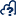Documentation

# Thermal search equivalent

The thermal search equivalent annotation addresses the question of whether it is worth going straight ahead on task in search of the next thermal or if it is worth diverting to find a stronger thermal. It does so by taking into account the present situation (i.e. location and altitude) and a set of assumptions about what the next thermal found might be (i.e. strength and relative location) as well as what is required to get there (e.g range, expected airmass). It will the plot a series of "equivalent" thermal strengths on the map, so that you can compare actual or possible decisions during the flight.What the "equivalence" plot actually shows is a set of thermal strengths that are equivalent for the given assumptions, i.e. a set of choices which would yield the same end result. The way to commonly use the annotation is to select a position in the flight using the glider cursor and then do one of the following:

• Select the parameters of the next thermal found in the flight and compare this to other possible or known options
• Specify an estimate for the next thermal in an assumed position and compare to how you actually faired
• Specify a known or assumed thermal and examine what the equivalent options would be if a diferent flight path was taken

In the example below we have selected a point in the flight at which the next thermal is of XX strength. The actual position of the thermal is marked by the light blue circle on the plot. We can then see the various colored segments indicating the thermal strengths that are equivalent for different deviations from the task. If we can find a thermal that is stronger than the number indicated, then this choice would be preferable to the thermal actually marked on the plot. If on we happen to find a thermal that is weaker than the strength indicated at that position, then this would represent a choice that is worse than the marked thermal.

The thermal search equivalent plot is based on a paper written by Johh Cochrane, which can be found here. It's basic conclusions are that the principal factor in deciding whether to deviate to find a better thermal is not the extra distance to be covered, but the angle of deviation from your target, assuming that there is sufficent altitude to make contact with a thermal.

## Creating an annotation

To create a thermal search equivalent annotation press thebutton on the map panel toolbar. This will automatically load the position and altitude of the current glider cursor fix into the calculation. You can then speficy the following parameters:

Glide to next thermal:

• LD - (Expected LD performance of glider - this only influences the range for which the equivalence plot will be drawn)
• Sink - The average sink that the glider will fly with to reach the destination thermal. This is not netto (i.e. atmospheric sink) but the combination of atmospheric and glider performance over the distance

Next thermal:

• Min contact height: The minimum heigth (QNH/MSL) at which you can be expected to find lift
• Average climb: the average climb of the next thermal
• Deviation angle: the deviation angle from the course to the next task point

The next thermal parameters are automatically copied from the next thermal found in the flight, but you may wish to override these by specifying other values.

You may also wish to reset the plot position to a new flight fix by moving the glider cursor to another fix and pressing thebutton. Please note that if you do so, the "next thermal" parameters will be reset as well.

You may create as many thermal search equivalent annotations as you like. To edit or delete an already created annotation, click on its marker button to reveal the annotation info popup and select the Edit button. You may then alter the annotation's parameters or press X to delete the annotation.